Kubojima, Y., Matsumura, Y., and Suzuki, Y. (2018). "Longitudinal vibration test for the use of a circular truncated cone as a log model of Japanese cedar," BioRes. 13(1), 1035-1041.

#### Abstract

The appropriate diameter ratio of log top and butt ends when using a round bar (cylinder shape) as a log model for estimating the density and Young’s modulus of a log was investigated. Square timbers of Japanese cedar (Cryptomeria japonica D. Don) with a length of 1200 mm were used as specimens and were machined into circular truncated cones. A longitudinal vibration test was performed to obtain the Young’s moduli of the square timbers and the circular truncated cones. The Young’s moduli were calculated using the circular truncated cone model and the round bar model. The density and Young’s modulus calculated by the circular truncated cone model were similar to those of the square timbers. Hence, it is considered that the circular truncated cone was effective for estimating the densities and Young’s moduli of logs. The density and Young’s modulus calculated by the round bar model differed from those of the square timbers when the diameters of the top ends were small. However, it is considered that the round bar can be used as a log model for actual logs.

Longitudinal Vibration Test for the Use of a Circular Truncated Cone as a Log Model of Japanese Cedar

Yoshitaka Kubojima,* Yukari Matsumura, and Youki Suzuki

The appropriate diameter ratio of log top and butt ends when using a round bar (cylinder shape) as a log model for estimating the density and Young’s modulus of a log was investigated. Square timbers of Japanese cedar (Cryptomeria japonica D. Don) with a length of 1200 mm were used as specimens and were machined into circular truncated cones. A longitudinal vibration test was performed to obtain the Young’s moduli of the square timbers and the circular truncated cones. The Young’s moduli were calculated using the circular truncated cone model and the round bar model. The density and Young’s modulus calculated by the circular truncated cone model were similar to those of the square timbers. Hence, it is considered that the circular truncated cone was effective for estimating the densities and Young’s moduli of logs. The density and Young’s modulus calculated by the round bar model differed from those of the square timbers when the diameters of the top ends were small. However, it is considered that the round bar can be used as a log model for actual logs.

Keywords: Circular truncated cone; Log; Longitudinal vibration test; Round bar; Young’s modulus

Contact information: Department of Wood Properties and Processing, Forestry and Forest Products Research Institute, 1 Matsunosato, Tsukuba, Ibaraki, 305-8687 Japan;

* Corresponding author: kubojima@ffpri.affrc.go.jp

INTRODUCTION

The elastic modulus of a timber specimen frequently must be determined using nondestructive testing methods before strength tests are conducted on structural elements and full-size structural timber. Such cases tend to involve vibration tests that are performed by tapping a specimen. Generally, a longitudinal vibration test is used, as well as a flexural vibration test (Sonoda 2014), and the longitudinal vibration test has been applied to logs.

According to the Japanese Agricultural Standard (JAS) for logs, a log is regarded as a round bar whose diameter is the average of the diameter of the top end and the diameter of the butt end (JAS 2016). However, the shape of a log typically can be better described as a circular truncated cone rather than a round bar. In this article the term “round bar” refers to a cylinder. It is a concern that a log may not be able to be regarded as a round bar when the difference between the diameter of the top end and that of the butt end is large.

The effects of the tapering of a log and the log diameter on the resonance frequency of the bending vibration of a tapered log have been studied by using a transfer-matix method (Sobue 1990). However, it cannot be said that the longitudinal vibration for the tapering logs has been sufficiently studied. Hence, whether or not the round bar can be used as a log model in the longitudinal vibration was examined.

EXPERIMENTAL

Theory

A circular truncated cone was prepared by rotating segment AB, shown in Fig. 1, about the x axis. The equation for the segment AB is: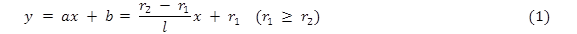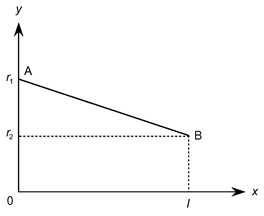Fig. 1. Segment AB, used to prepare a circular truncated cone

The cross-sectional area is: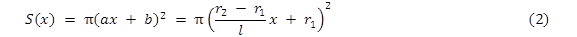The differential equation for longitudinal vibration of the circular truncated cone having radii r1 and r2 and length l is,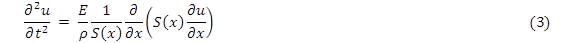where xu(xt), tE, and ρ are the distance along the bar, the longitudinal displacement, time, Young’s modulus, and density, respectively. Solving Eq. 3 results in Eq. 4,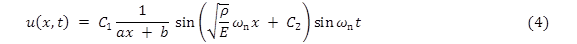where C1 and C2 are constants, ω (equal to 2πf, where f is the resonance frequency) is the angular frequency, and the subscript n is the mode number.

For a free-free condition,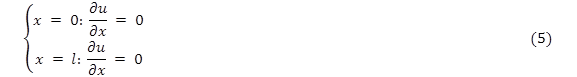Thus, the following frequency equation can be obtained,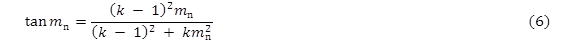where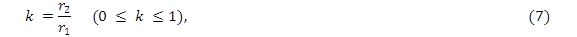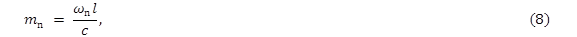and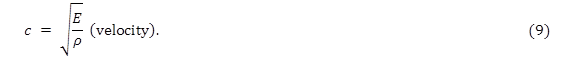The Young’s modulus can be determined as follows: Substituting the experimental k value into Eq. 6 gives mn. In this study, given various k values, Eq. 6 was solved using Mathematica (version 10.4J, Wolfram Research, Champaign, IL, USA) software. Substituting the calculated mn, the measured resonance frequency, and the measured length into Eq. 8 gives c. Substituting the calculated c, the measured dimensions, and the measured mass into Eq. 9 gives the Young’s modulus.

The volume of the circular truncated cone is: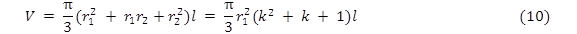Then, the Young’s modulus of the circular truncated cone is,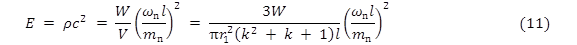where W is weight.

If S(x) is constant, then Eq. 3 is as follows: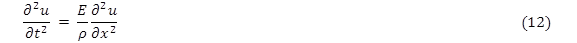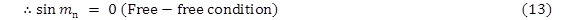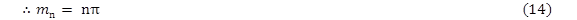Substituting k = 1 (r1 = r2) into Eq. 6 gives the following equation: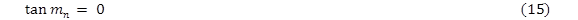Equation 15 also gives Eq. 14. The Young’s modulus is calculated by the following equation: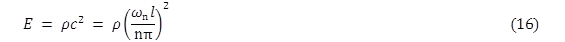In contrast, the volume of the round bar via the JAS method is: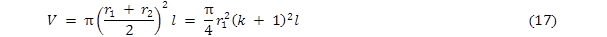Then, the Young’s modulus of the round bar is: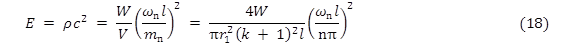Materials

Air-dried Japanese cedar (Cryptomeria japonica D. Don.) squared lumber without pith was used for the specimens, which were 1200 mm (longitudinal direction) 110 mm (radial direction) 110 mm (tangential direction). Seven clear specimens were used. Circular truncated cones with the following combinations of diameters d1 and d2 (in mm) were prepared from the square timber: (d1d2) = (100, 30), (100, 40), (100, 50), (100, 60), (100, 70), (100, 80), and (100, 90). The length of the circular truncated cones was 1000 mm.

The specimens were conditioned at 20 °C and 65% relative humidity (R. H.) until their weight was constant. The tests were conducted under 20 °C and 65% R. H.

Methods

Longitudinal vibration test

To obtain the Young’s modulus, free-free longitudinal vibration tests were conducted using the following procedure: The test bar was placed on a small sponge near its center point. Vibration was initiated in the longitudinal direction at one end with a hammer, while the first-mode vibration of the specimen was detected with a microphone at the other end. The signal was processed through a fast Fourier transform (FFT) digital signal analyzer (Multi-Purpose FFT Analyzer CF-5220, Ono-Sokki, Co., Ltd., Yokohama, Japan) to observe high-resolution resonance frequencies. A diagram of the experimental setup is shown in Fig. 2.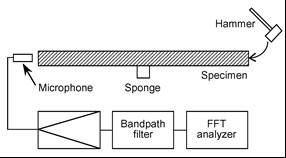Fig. 2. Diagram of the experimental setup

RESULTS AND DISCUSSION

The densities and Young’s moduli of the square timbers are shown in Table 1. The Young’s moduli were obtained from Eq. 16.

Table 2 shows the densities and Young’s moduli of the circular truncated cones. They were calculated by the circular truncated cone model and round bar model. The density and Young’s modulus calculated by the circular truncated cone model are expressed by ρCTC and ECTC, respectively. The density and Young’s modulus calculated by the round bar model are expressed by PRB and ERB, respectively. The density and Young’s modulus of the square timbers are expressed by ρST and EST, respectively.

Table 1. Densities and Young’s Moduli of the Square Timbers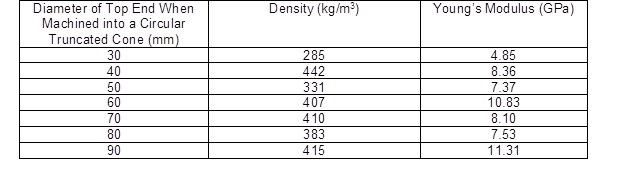The ratios ρCTC / ρST and ECTC / EST were around 1. Since wood properties are not significantly changed by machining square timbers to circular truncated cones, CTC and ECTC will be almost equal to ρST and EST, respectively. Hence, the accurate density and Young’s modulus of the circular truncated cone could be obtained by the developed circular truncated cone model. Thus, it is considered that the circular truncated model will be effective for estimating the density and Young’s modulus of logs.

However, the ratios ρRB / ρST and ERB / EST increased as the diameters of the top ends decreased. Hence, it is apparent that the accurate density and Young’s modulus of the circular truncated cone cannot be obtained by the traditional round bar model.

Table 2. Densities and Young’s moduli of the Circular Truncated Cones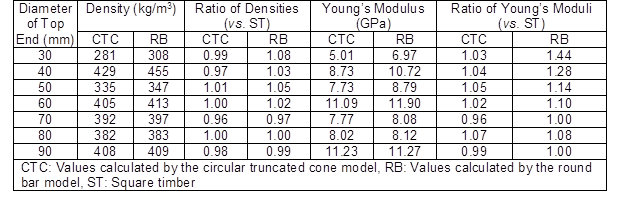Still the round bar model will be effective for estimating the density and Young’s modulus of logs. When the circular truncated cone is used as a log model, it is necessary to solve Eq. 6 for each k. Because solving Eq. 6 for each log is not easy, using the round bar model is suitable for the actual cases.

A relationship between the ratio of densities and k, and a relationship between the ratio of Young’s moduli and k, are considered.

The ratio of densities ρRB / ρCTC is expressed by the following equation using Eqs. 10 and 17: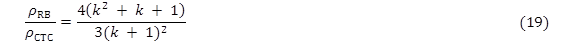The ratio of Young’s moduli of the first mode ERB / ECTC is expressed by the following equation using Eqs. 11 and 18: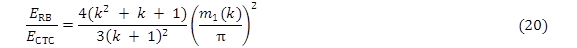The ratios ρRB / ρCTC and ERB / ECTC were plotted against k, as shown in Fig. 3. For example, for ρRB / ρCTC ≤ 1.1, k ≥ 0.3; and for ρRB / ρCTC ≤ 1.05, k ≥ 0.45. For ERB / ECTC ≤ 1.1, k ≥ 0.55; and for ERB / ECTC ≤ 1.05, k ≥ 0.66. Given the estimation accuracy at each site, which are ρRB / ρCTC and ERB / ECTC, the range of k to apply the round bar model to logs can be obtained from Fig. 3.

Several reports have been published with data for the diameters of the top ends and the butt ends of Japanese cedar wood (Matsumura et al. 2013; Matsumura 2014; Goto et al. 2017). Because many samples were used in Matsumura’s research (2014), the corresponding data were used here. The diameters of the top ends and the butt ends are shown in Table 3. The minimum, average (standard deviation), and maximum k for the logs used in Matsumura (2014), and shown in Table 3, are 0.72, 0.88 (0.042), and 0.95, respectively, for the large diameter logs and 0.76, 0.87 (0.036), and 0.95, respectively, for the middle diameter logs. For k = 0.72, ρRB / ρCTC and ERB / ECTC were 1.01 and 1.03, respectively. These results suggested that the round bar can be used as a log model to estimate the densities and Young’s moduli of actual logs.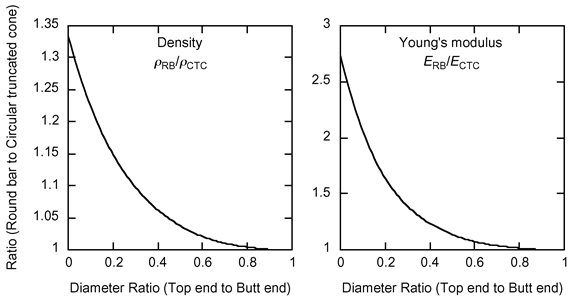Fig. 3. Relationships of ρRB / ρCTC vsk and ERB / ECTC vsk

Table 3. Diameters of the Top Ends and the Butt Ends of Japanese Cedar (Matsumura 2014)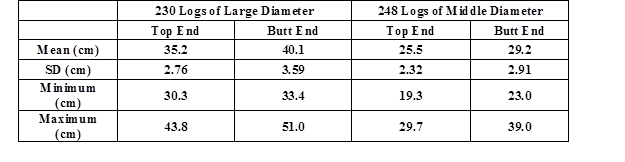CONCLUSIONS

1. The ratios ρCTC / ρST and ECTC / EST were approximately 1. Hence, it was considered that the circular truncated cone was effective for estimating the densities and Young’s moduli of the logs.
2. The ratios ρRB / ρST and ERB / EST increased as the diameters of the top ends decreased.
3. It was considered that a round bar (cylinder) could be used as a log model for actual logs.

REFERENCES CITED

Goto, T., Nakayama, S., and Furuno, T. (2017). “Comparison of wood qualities and strength properties between pithless timber and boxed heart timber of sugi planted in Shimane prefecture: Bending performances of square timber and flat square timber,” Wood Industry 72(7), 262-267.

Japanese Agricultural Standard (JAS) (2016). “Forestry products: Log,” Japanese Agricultural Standards Association, Tokyo, Japan.

Matsumura, Y. (2014). “Log specifications and wood properties of large-diameter logs,” Wood Industry 69(11), 486-491.

Matsumura, Y., Ikami, Y., Murata, K., and Matsumura, J. (2013). “Quality of squared lumber without pith sawn from large-diameter sugi (Cryptomeria japonica) logs,” Mokuzai Gakkaishi 59(3), 138-145.

Sobue, N. (1990). “Correlation factors of the resonance frequency for tapering and shear deformation of a log in flexural vibration,” Mokuzai Gakkaishi 36(9), 760-764.

Sonoda, S. (2014). “Acoustic vibrations and their higher harmonics for non-destructive measurement techniques,” Wood Industry 69(4), 183-186.

Article submitted: September 5, 2017; Peer review completed: November 18, 2017; Revised version received and accepted: December 5, 2017; Published: December 11, 2017.

DOI: 10.15376/biores.13.1.1035-1041